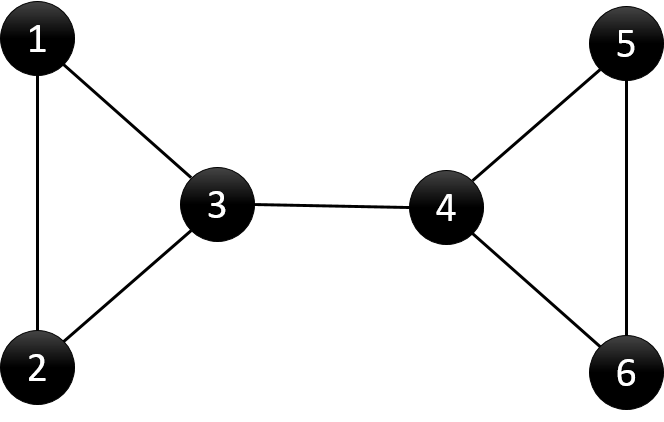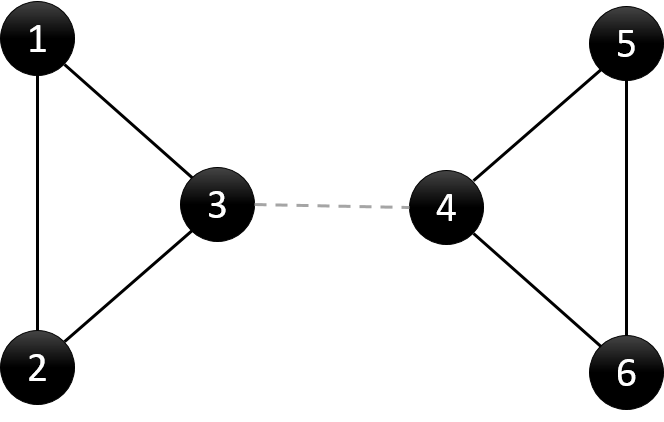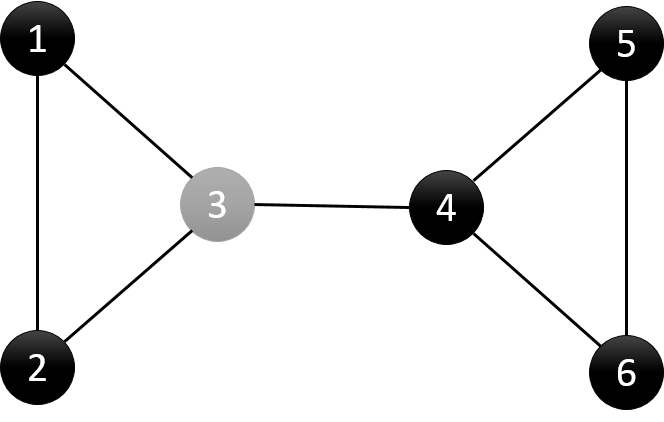# hihoCoder week 52-1-连通性·一

hihoCoder week 52-1-连通性·一6 7
1 2
1 3
2 3
3 4
4 5
4 6
5 6

3 4
3 4```#include<iostream>
#include<cstdio>
#include<set>
#include<algorithm>
#include<vector>
using namespace std;
const int kMaxN = 20005;
int n, m;
typedef struct edge
{
int x, y;
bool operator<(const edge& p)const//如果要加入到set中，需要重载<
{
return (this->x<p.x) || ((this->x == p.x) && (this->y<p.y));
}
};
set<int> points;
set<edge> edges;
vector<vector<int>> graph;
int visit[kMaxN];
int dfn[kMaxN];
int low[kMaxN];
int parent[kMaxN];
void dfs(int u) {
//记录dfs遍历次序
static int counter = 0;
//记录节点u的子树数
int children = 0;
visit[u] = 1;
//初始化dfn与low
dfn[u] = low[u] = ++counter;
for (int i = 0; i < graph[u].size();i++) {
int v = graph[u][i];
//节点v未被访问，则(u,v)为树边
if (visit[v]==0) {
children++;
parent[v] = u;
dfs(v);
low[u] = min(low[u], low[v]);
//case (1)
if (parent[u] == 0 && children > 1) {
points.insert(u);
}
//case (2)
if (parent[u] != 0 && low[v] >= dfn[u]) {
points.insert(u);
}
//bridge
if (low[v] > dfn[u]) {
edge e;
e.x = u;
e.y = v;
if (u > v)
{
e.x = v;
e.y = u;
}
edges.insert(e);
}
}
//节点v已访问，则(u,v)为回边
else if (v != parent[u]) {
low[u] = min(low[u], dfn[v]);
}
}
}
int main()
{
scanf("%d %d", &n, &m);
graph.resize(n + 1);
int u, v;
while (m--)
{
scanf("%d %d", &u, &v);
graph[u].push_back(v);
graph[v].push_back(u);
}
dfs(1);
if (points.size() == 0)
printf("Null\n");
else
{
set<int>::iterator it = points.begin();
while (it != points.end())
{
printf("%d ", *it);
it++;
}
printf("\n");
}
if (edges.size() > 0)
{
set<edge>::iterator it = edges.begin();
while (it != edges.end())
{
printf("%d %d\n", (*it).x, (*it).y);
it++;
}
}
return 0;
}
```

This site uses Akismet to reduce spam. Learn how your comment data is processed.